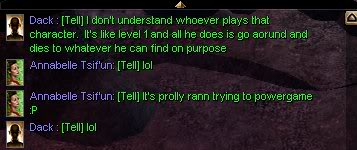Anphillia ExodusNWN 1 Faction roleplay PW using CEP v2.3HomeFAQSearchMemberlistUsergroupsRegisterLog in

 Anphillia Exodus :: Changes and Fixes :: Classes :: Bard Share

Curse SongAuthorMessage

StaffJoin date : 2009-04-29
Location : UKSubject: Curse SongWed Apr 29, 2009 3:58 am Curse Song on Anphillia has been improved. Here is how it works now:Quote :Class Level = 40Perform Rank = 100Attack = 4Damage = 3Will = 4Fortitude = 4Reflex = 5HP = 60AC = 8Skill Penalty = 22Quote :Class Level = 39Perform Rank = 95Attack = 3Damage = 3Will = 3Fortitude = 2Reflex = 3HP = 50AC = 7Skill Penalty = 19Quote :Class Level = 30Perform Rank = 90Attack = 2Damage = 3Will = 3Fortitude = 2Reflex = 2HP = 48AC = 7Skill Penalty = 18Quote :Class Level = 29Perform Rank = 90Attack = 2Damage = 3Will = 3Fortitude = 2Reflex = 2HP = 46AC = 6Skill Penalty = 17Quote :Class Level = 28Perform Rank = 90Attack = 2Damage = 3Will = 3Fortitude = 2Reflex = 2HP = 44AC = 6Skill Penalty = 16Quote :Class Level = 27Perform Rank = 85Attack = 2Damage = 3Will = 3Fortitude = 2Reflex = 2HP = 42AC = 6Skill Penalty = 15Quote :Class Level = 26Perform Rank = 80Attack = 2Damage = 3Will = 3Fortitude = 2Reflex = 2HP = 40AC = 6Skill Penalty = 14Quote :Class Level = 25Perform Rank = 75Attack = 2Damage = 3Will = 3Fortitude = 2Reflex = 2HP = 38AC = 6Skill Penalty = 13Quote :Class Level = 24Perform Rank = 70Attack = 2Damage = 3Will = 3Fortitude = 2Reflex = 2HP = 36AC = 5Skill Penalty = 12Quote :Class Level = 23Perform Rank = 65Attack = 2Damage = 3Will = 3Fortitude = 2Reflex = 2HP = 34AC = 5Skill Penalty = 11Quote :Class Level = 22Perform Rank = 60Attack = 2Damage = 3Will = 3Fortitude = 2Reflex = 2HP = 32AC = 5Skill Penalty = 10Quote :Class Level = 21Perform Rank = 55Attack = 2Damage = 3Will = 3Fortitude = 2Reflex = 2HP = 30AC = 5Skill Penalty = 9Quote :Class Level = 20Perform Rank = 50Attack = 2Damage = 3Will = 3Fortitude = 2Reflex = 2HP = 28AC = 5Skill Penalty = 8Quote :Class Level = 19Perform Rank = 45Attack = 2Damage = 3Will = 3Fortitude = 2Reflex = 2HP = 26AC = 5Skill Penalty = 7Quote :Class Level = 18Perform Rank = 40Attack = 2Damage = 3Will = 3Fortitude = 2Reflex = 2HP = 24AC = 5Skill Penalty = 6Quote :Class Level = 17Perform Rank = 35Attack = 2Damage = 3Will = 3Fortitude = 2Reflex = 2HP = 22AC = 5Skill Penalty = 5Quote :Class Level = 16Perform Rank = 30Attack = 2Damage = 3Will = 3Fortitude = 2Reflex = 2HP = 20AC = 5Skill Penalty = 4Quote :Class Level = 15Perform Rank = 24Attack = 2Damage = 3Will = 2Fortitude = 2Reflex = 2HP = 16AC = 4Skill Penalty = 3Quote :Class Level = 14Perform Rank = 21Attack = 2Damage = 3Will = 1Fortitude = 1Reflex = 1HP = 16AC = 3Skill Penalty = 2Quote :Class Level = 12Perform Rank = 18Attack = 2Damage = 2Will = 1Fortitude = 1Reflex = 1HP = 8AC = 2Skill Penalty = 2Quote :Class Level = 8Perform Rank = 15Attack = 2Damage = 2Will = 1Fortitude = 1Reflex = 1HP = 8AC = 0Skill Penalty = 1Quote :Class Level = 6Perform Rank = 12Attack = 1Damage = 2Will = 1Fortitude = 1Reflex = 1HP = 0AC = 0Skill Penalty = 1Quote :Class Level = 3Perform Rank = 9Attack = 1Damage = 2Will = 1Fortitude = 1Reflex = 0HP = 0AC = 0Skill Penalty = 0Quote :Class Level = 2Perform Rank = 6Attack = 1Damage = 1Will = 1Fortitude = 0Reflex = 0HP = 0AC = 0Skill Penalty = 0Quote :Class Level = 1Perform Rank = 3Attack = 1Damage = 1Will = 0Fortitude = 0Reflex = 0HP = 0AC = 0Skill Penalty = 0_________________Anphillia Exodus; Gameworld Page - Vote nicely!Curse SongPage 1 of 1
 Similar topics» Kentucky Rain - The Rooster Song - Video!